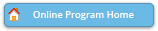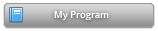#### Abstract Details

 Activity Number: 509 - Statistical Methodology Type: Contributed Date/Time: Wednesday, July 31, 2019 : 10:30 AM to 12:20 PM Sponsor: IMS Abstract #305270 Presentation Title: Sample Size Calculations in Simple Linear Regression: Exact Approach Author(s): Marepalli Rao* and Tianyuan B Guan Companies: University of Cincinnati and University of Cincinnati Keywords: Sample Size; Linear Regression; Level; Power; Effect Size; Exact Distribution Abstract: The simple linear regression is a five-parameter model involving a numeric response variable and a lone predictor. Testing the nullity of the slope parameter of the model was never a problem. The conditional test based on the least squares estimator of the slope parameter conditioned on the data of the predictor is amply adequate. However, sample size calculation for given level, power, and alternative value of the slope parameter is fraught with difficulties. The predictor is throwing a wrench in the calculations. We counter this problem by simply finding the exact distribution of the least squares estimator of the slope parameter unconditioned in every way. An alternative to the classical testing problem of the slope parameter, we could move on to testing the nullity of the correlation coefficient between the response variable and predictor. We will demonstrate that this could solve the problem and the sample size that comes out should work out for our original sample size calculation problem.

Authors who are presenting talks have a * after their name.